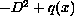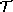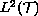Electron. J. Diff. Eqns., Vol. 1997(1997), No. 23, pp 1-30.

### Hill's equation for a homogeneous tree Robert Carlson

Abstract:
The analysis of Hill's operatorforeven and periodic is extended from the real line to homogeneous trees. Generalizing the classical problem, a detailed analysis of Hill's equation and its related operator theory onis provided. The multipliers for this new version of Hill's equation are identified and analyzed. An explicit description of the resolvent is given. The spectrum is exactly described when the degree of the tree is greater than two, in which case there are both spectral bands and eigenvalues. Spectral projections are computed by means of an eigenfunction expansion. Long time asymptotic expansions for the associated semigroup kernel are also described. A summation formula expresses the resolvent for a regular graph as a function of the resolvent of its covering homogeneous tree and the covering map. In the case of a finite regular graph, a trace formula relates the spectrum of the Hill's operator to the lengths of closed paths in the graph.

Submitted August 24, 1997. Published December 18, 1997.
Math Subject Classification: 34L40.
Key Words: Spectral graph theory, Hill's equation, periodic potential.

Show me the PDF file (254 KB), TEX file, and other files for this article.

Robert Carlson